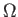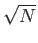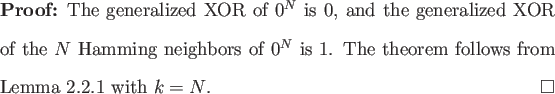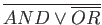Next: Determining the Oracle String Up: A Lemma for Proving Previous: A Lemma for Proving   Contents

## Application to Generalized XOR

To see how simple proofs can be with Lemma 2.2.1 we provide an example. Generalized XOR is the N-bit Boolean function that is 0 if and only if its input bits are all the same.

Theorem 2.2.1() oracle queries are required to compute the generalized XOR of N bits in the bounded error setting.This lower bound is asymptotically tight: Beals et al. provide O() oracle query algorithms for computing the AND or OR of N bits in the bounded error setting , and the generalized XOR of N bits is just. Unfortunately, Ambainis' Theorem does not always perform this well, as we demonstrate in the next two sections.Next: Determining the Oracle String Up: A Lemma for Proving Previous: A Lemma for Proving   Contents
Matthew Hayward Lower Query Bounds in the Quantum Oracle Model GitHub Repository